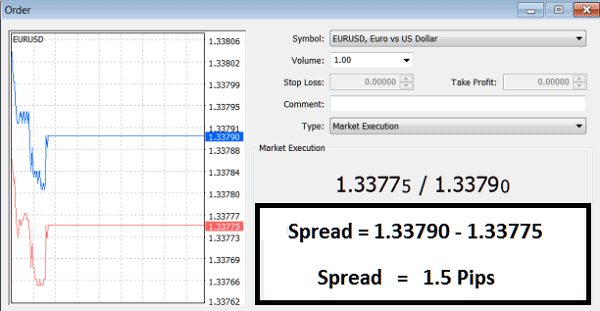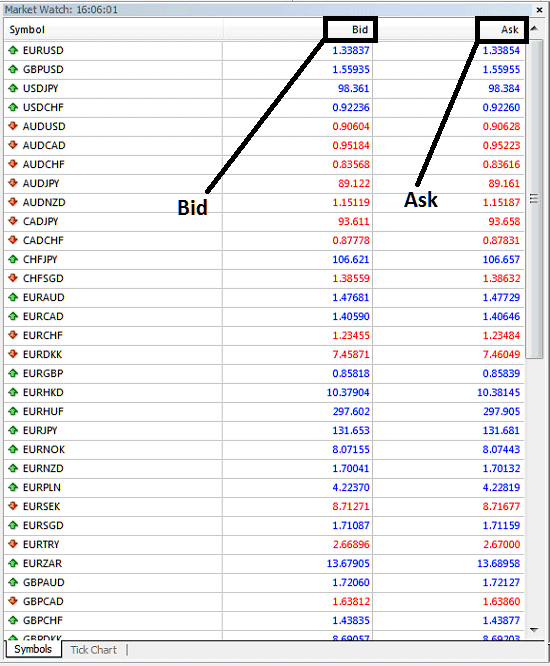# Forex Lots and Contracts - Explain Lot Size, Leverage and Margin and Spread - Bid and Ask Price

## Forex Lots and Contracts Explained - Lot Size Forex Explained - Standard, Mini and Micro Lot

Forex is traded using Standard Lots. Lot Size Forex - One standard forex lot is equal to 100,000 units of currency. One standard forex lot is also known as a one forex contract.

The Forex Lot or Contract is the size of the amount of currency that is to be bought or sold in the online exchange market by a forex trader.

Lot Size Forex - The Standard Forex Lot which is equal to \$100,000 Dollars worth of currency is not traded physically, but this \$100,000 Dollar amount is represented by a forex contract.

The two terms, that is 1 Standard Lot Forex and 1 Contract Forex can be used interchangeably, because both of them refer to the same thing - \$100,000 Dollars of Forex Trade Transaction in the Forex Market.

Why Trade Forex in Units of Currencies of \$100,000

The reason why such large forex contracts are used in forex trading is so as to increase the value of a forex pip (profit).

The Currency Price moves are measured in Forex Pips. PIP - Price Interest Points

100 Forex Pips Make 1 Cent, therefore the forex price movements are calculated using very small price movements.

EURUSD currency pair will be quoted as 1.3452

The last decimal is the Forex PIP (4th Decimal Place)

In forex trading, a fraction of a pip movement was introduced by forex trading brokers and this is now referred to as fractional pips or pipettes.

EURUSD currency pair will be quoted as 1.34520

The last decimal in the forex currency quote is the PIPETTE (5th Decimal Place)

To answer the question why forex currencies are traded in forex lots of \$100, 000 Units of a currency, we shall use the following forex trading example to explain:

Most currencies in the forex market will not even move more than 1 Cents a Day, 1 Cent is equal to 100 Forex Pips, and the most common price moves in forex trading are between 40 pips and 80 Pips.

Example:

• For one forex contract (standard lot forex) of \$100,000

Value of 1 forex pip = 0.0001 USD

If one unit is used of 1 Euro then Value of 1 pip = 0.0001 USD

If 100,000 units (1 Forex contract) of Euros are used then value of 1 forex pip = 100,000*0.0001 = 10 USD

NB: 100,000 units of Euro are used but the profit is calculated in USD, the quote currency and because the Forex Exchange Trade is EURUSD.

Therefore you can see that if you are trading with only 1 Euro your profit per 1 PIP forex price movement would have been 0.0001 USD, not even equal to 1 Cent, But when you forex trade with a standard forex lot of 100,000 Units of currency then the profit is equal to \$10 dollars per 1 Forex PIP.

This is why Forex is traded using 100, 000 Units of Currency Contracts/Lots.

## But How Can any Forex Trader afford \$100,000 to Invest With?

That is a very good question, the answer is Forex LEVERAGE and MARGIN

You don't need \$100,000 Dollars to trade forex, with leverage and margin you only need \$1,000 dollars to transact a forex contract, but how?

We shall explain using the forex trading example below:

In Forex, a small deposit can control a much larger transaction this is called Leveraging, which gives the forex traders the ability to make nice profits, and at the same time keep risk capital to a minimum. The forex trader will transact on borrowed capital, having \$1,000 dollars a forex trader can borrow the rest using a leverage option such as 100:1 - meaning that the forex trader borrows 100 dollars for every 1 dollar they have, therefore in total they will control a total of \$100,000 dollars after using forex leverage of 100:1 - this is how forex leverage works.

Forex Leverage is expressed in the form of a ratio, for Example forex leverage 100:1, means the forex broker with give a forex trader \$100 Dollars for every 1 dollar that the trader has in their forex trading account.

Forex Margin is the amount of money required by your forex broker so as to allow you to continue trading forex with the leveraged amount. Forex Margin is the amount you deposit so as to open a forex trading account with. If you deposit \$1,000 in your forex trading account - then that is your forex margin.

With forex leverage it is possible for retail investors and retail forex traders to trade the forex market. Forex Leverage of 100:1 means that for every dollar you deposit, the forex broker will give you 100 dollars. This also means that in converse the forex broker requires you to maintain a margin of \$1 Dollar for every \$100 Dollars that they give you so as to let you continue controlling the borrowed amount of capital that they have given you for forex trading.

Forex Margin Trading Example:

If you deposit \$1000 in your forex trading account, and the forex broker gives you leverage of 100:1 then it means you now have \$1,000*(100) = \$100,000 Dollars that you can forex trade with.

Because the total amount you control is \$100,000 and your money is \$1,000 which is 1%, this will mean your forex margin requirement is 1 %.

A forex broker can tell you that our margin forex account requires 1 % margin - which means their leverage is 100:1, if the forex broker tells you their margin forex account require 2 % margin it means their forex leverage is 50:1 (leverage 50:1 - calculation: 1 is 2 % of 50 when using 50:1 forex leverage ratio).

Therefore, with Forex Leverage and Margin as shown above forex traders are not required to deposit all the cash for the whole forex trade position they are going to trade. The forex trading account they open will therefore be referred to as a Forex Margin Account meaning they are trading on margin - the funds in their forex account is the margin for the forex leverage they are using for trading.

## Spread - Forex Spreads - What is Forex Spread?

The forex spread is the difference between the price which you buy and the price that the forex broker is offering to sell.

What is Forex Spread? - Forex Spread can also be defined as the difference between the Bid Price and the Ask Price, this forex Bid price and Ask price are shown on the example below and the forex spread is also calculated as the difference between these 2 price points.

Example of Forex Spreads on MT4 - What is Forex Spread?EURUSD Currency Pair - Bid Ask Spread Calculation of 1.5 Forex Pips - What is Spread?Example of How to Calculate Forex Spread:

If The Bid Ask Quote of EURUSD is 1.2914/1.2917

The Forex Spread is 1.2917-1.2914 = 3 pips

These 3 forex pips is profit for the forex broker. If you were to buy EURUSD you would buy at 1.2917. If you wanted to sell back the EURUSD you have just bought, the forex broker will buy from you at 1.2914, i.e. 3 pips less than the forex price you bought, the forex broker makes profit this way. The low spreads charged is about 2 pips for major forex currency pairs.

Update: there was introduction of fractional pips, with fractional pips forex brokers also argued that they could now offer lower spreads - spread such as 1.8 pips or 1.7 pips instead of 2 pips forex spreads.

For Example in the above spread illustrated on the MetaTrader 4 Forex Platform Bid Ask Forex Quotes the spread is:

1.33790/1.33750

Spread is 1.33790-1.33750= 1.5 Pips

Most forex brokers now use the fractional pips, 5 Decimal Place Forex Quotes so as to offer forex traders lower forex spreads, commonly referred to as "tight spreads", for example 1.8 Pips Spread instead of fixed 2 Pips forex spreads.

Examples of Average Spreads (Major Pairs) in The Forex Market

• EURUSD - 2.0 Pips Forex Spread
• USDJPY - 2.0 Pips Forex Spread

• GBPUSD - 3.2 Pips Forex Spread

• USDCHF - 3.2 Pips Forex Spread

Bid is the price at which you sell

Ask is the price at which you buy

If the bid ask quote for EURUSD is 1.29140/1.29170

Therefore:

Bid Price =1.29140

Example Of Bid Ask on MetaTrader 4 - Bid Ask Price MT4 ExampleBid Ask Prices of Various Forex Currencies on MT4 - Bid Ask Price Explained

### Forex Mini Lots and Forex Micro Lots - Mini Lot vs Micro Lot

As a note, there is also the fraction of 1 Forex Standard Lot, these fractions of the standard forex lots are provided by forex brokers so as to make Forex more affordable to forex traders with minimum capital required to trade forex micro lots being as little as \$5 dollars.

The Fraction of a Standard forex lot is called Mini Forex Lot which is 1/10 of a standard forex lot and Micro Forex Lot which is 1/100 of a Standard Forex Lot

Mini Lot Forex Lot = \$10, 000 Units of Currency

Micro Lot Forex Lot = \$1, 000 Units of Currency

These forex micro lots were introduced to make the forex trading market more accessible to the retail forex investors and retail forex traders as well as attract more and more retail forex traders and retail investors. Maybe this is why Forex has become very popular, even with as little as \$100 dollars a beginner forex trader can enter this online forex trading market and start trading forex online.